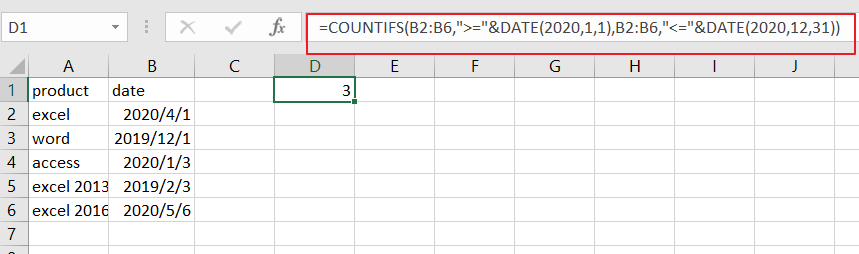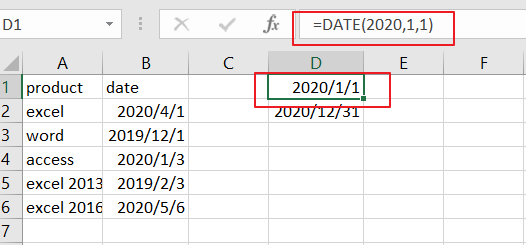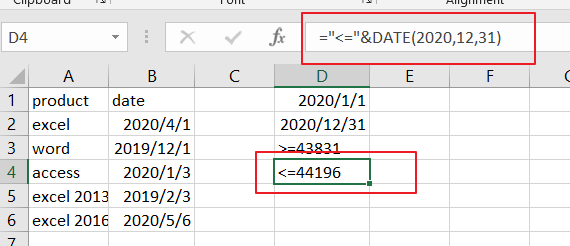# How to Count Cells between Two Dates or Times in Excel

This post will guide you how to count cells between two dates using a formula in Excel 2013/2016. How do I count times between a given time or date range based on a condition in Excel. Is there a easy way to count cells between two given dates in a selected range of cells in Excel.

## Count Cells between Dates

Assume that you have a list of data in range A1:B6, and you want to count cells which between 2020/1/1 and 2020/12/31. In the below steps, and you should learn that how can we use COUNTIF function to achieve the result of counting times between two given dates in Excel.

You can use the following formula based on the COUNTIF function:

=COUNTIFS(B2:B6,”>=”&DATE(2020,1,1),B2:B6,”<=”&DATE(2020,12,31))Let’s see how this formula works:

The COUNTIFS function can be used to count cells that meet one or more criteria in the given range cells.

From the above screenshot, and you can see that this formula will count sales date in the year 2020, that appear in the range B2:B6. To count cells between two dates, and you need to provide two criteria in COUNTIFS formula. One is for start date and one is for the end date. And the range of cells that you want to compare is B2:B6.

And you still need to construct both starting date and ending date for comparing in the above COUNTIFS formula. You can use the DATE function to do it. Like below:

=DATE(2020,1,1)

=DATE(2020,12,31)You also need to concatenate “greater than or equal to” or “smaller than or equal to” operators with concatenate operator to build those two conditions.

=”>=”&DATE(2020,1,1)

=”<=”&DATE(2020,12,31)Then you can combine these formulas into one like as below:

= B2:B6,”>=”&DATE(2020,1,1),B2:B6,”<=”&DATE(2020,12,31)

This formula can be added into the COUNTIFS formula as its criteria. It performs AND logic because it must be satisfied for both two conditions.

### Related Functions

• Excel COUNTIFS function
The Excel COUNTIFS function returns the count of cells in a range that meet one or more criteria. The syntax of the COUNTIFS function is as below:= COUNTIFS(criteria_range1, criteria1, [criteria_range2, criteria2]…)…
• Excel DATE function
The Excel DATE function returns the serial number for a date.The syntax of the DATE function is as below:= DATE (year, month, day)…